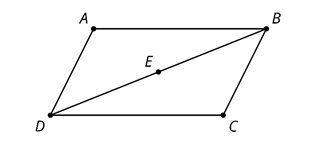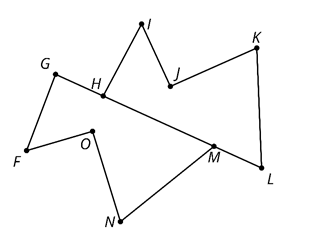# G.2.2.3 Are These Parts Congruent?

Triangle ABD is a rotation of triangle CDB around point E by 180 degrees. Is angle ADB congruent to angle CDB? If so, explain your reasoning. If not, which angle is ADB congruent to?Polygon HIJKL is a reflection and translation of polygon GFONM. Is segment KJ congruent to segment NM? If so, explain your reasoning. If not, which segment is NM congruent to?Quadrilateral PQRS is a rotation of polygon VZYW. Is angle QRS congruent to angle ZYW? If so, explain your reasoning. If not, which angle is QRS congruent to? (No picture on this one - just use the order of the letters to answer the question)